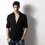MatheMagic !!!

Two boys went to a book shop and purchased a book which costs $50. They paid$25 each for the book and left the store. When the store manager went to the cashier, he found that they have taken $5 extra from them. So, the actual cost of the book was$45. The manager gave $5 to a worker out there to return it to those boys. Let's make it more interesting, the worker was a bit greedy, he took$2 from it and returned $3 to the boys. They distributed$1.5 and $1.5 each and thought that they have paid$25 - $1.5 =$23.5 each for the book. Here comes the problem, Now, we all (I mean those who are reading this thread) know that the boys paid $23.5 +$23.5 = $47 and the worker have only$2 which adds up to $49. Where do you think$1 lost in the process ?Note by Abhishek Kumar
6 years, 4 months ago

This discussion board is a place to discuss our Daily Challenges and the math and science related to those challenges. Explanations are more than just a solution — they should explain the steps and thinking strategies that you used to obtain the solution. Comments should further the discussion of math and science.

When posting on Brilliant:

• Use the emojis to react to an explanation, whether you're congratulating a job well done , or just really confused .
• Ask specific questions about the challenge or the steps in somebody's explanation. Well-posed questions can add a lot to the discussion, but posting "I don't understand!" doesn't help anyone.
• Try to contribute something new to the discussion, whether it is an extension, generalization or other idea related to the challenge.
• Stay on topic — we're all here to learn more about math and science, not to hear about your favorite get-rich-quick scheme or current world events.

MarkdownAppears as
*italics* or _italics_ italics
**bold** or __bold__ bold

- bulleted
- list

• bulleted
• list

1. numbered
2. list

1. numbered
2. list
Note: you must add a full line of space before and after lists for them to show up correctly
paragraph 1

paragraph 2

paragraph 1

paragraph 2

> This is a quote
This is a quote
# I indented these lines
# 4 spaces, and now they show
# up as a code block.

print "hello world"
# I indented these lines
# 4 spaces, and now they show
# up as a code block.

print "hello world"
MathAppears as
Remember to wrap math in $$...$$ or $...$ to ensure proper formatting.
2 \times 3 $2 \times 3$
2^{34} $2^{34}$
a_{i-1} $a_{i-1}$
\frac{2}{3} $\frac{2}{3}$
\sqrt{2} $\sqrt{2}$
\sum_{i=1}^3 $\sum_{i=1}^3$
\sin \theta $\sin \theta$
\boxed{123} $\boxed{123}$

Sort by:

you are supposed to add $3 to$47 because the worker's $2 is counted in the$47

- 6 years, 4 months ago

u r talking about cost price in the first half and then reverse calculating it by sell price in the second half.so its obvious why they are not adding up

- 6 years, 4 months ago

that is subtract $3 from$50 then subtract $2 thus it adds up - 6 years, 4 months ago Log in to reply do like this they each have paid$23.5 so in total $47.now waht u r duin rong is that they actually bought the book for$45(after gettin money back) but they paid $47 because the cashier cheated$2 on them i.e $47 -$2

- 6 years, 4 months ago